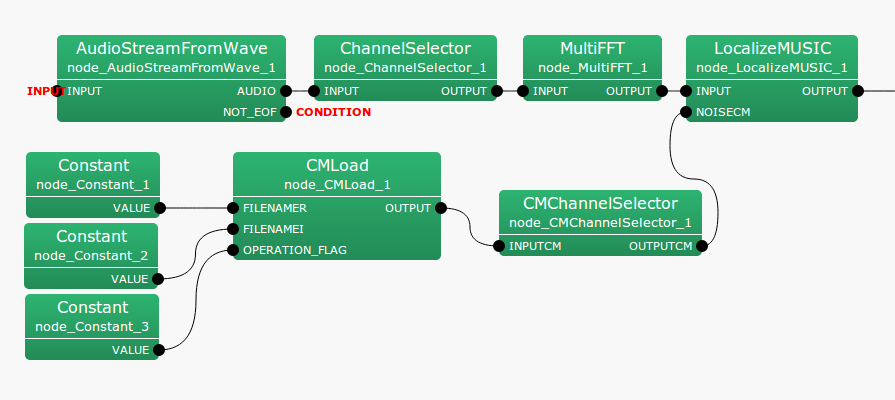## 6.2.3 CMChannelSelector

### 6.2.3.1 Module Overview

From a multi-channel correlation matrix, get only the specified channel data in the specified sequence.

None.

### 6.2.3.3 Usage

In what case is the node used?

From the multi-channel correlation matrix that was input, using this when channels not required are to be deleted, or the channel sequence is to be exchanged, or when the channel is to be copied.

Typical Examples

Figure. 6.19 shows a usage example for the CMChannelSelector  node. The input terminal is connected to a correlation matrix calculated from CMMakerFromFFT  or CMMakerFromFFTwithFlag , etc. (type is Matrix<complex<float> >  type, but to handled a correlation matrix, convert the three dimensional complex array to a two dimensional complex array and then output).Figure 6.19: Network Example using CMChannelSelector

### 6.2.3.4 I/O and property setting of the node

Table 6.19: Parameter list of CMChannelSelector
 Parameter Type Default Unit Description SELECTOR > Specifies the channel numbers to be output

Input

INPUTCM

: Matrix<complex<float> >  type. Correlation matrix for each frequency bin. $M$-th order complex square array with correlation matrix inputs $NFFT/2 + 1$ items. Matrix<complex<float> >  indicates the row of frequency ($NFFT/2 + 1$ rows), and column of complex correlation matrix ($M * M$ columns).

Output

OUTPUTCM

: Matrix<complex<float> >  type. Same as INPUTCM.

Parameter

SELECTOR

: Vector<int>  type. No default value (<Vector<int> >). Specifies the channel numbers to be used. Channel numbers start from 0.

Example: From 5 channels (0-4), specify <Vector <int> 2 3 4> when using only channels 2, 3, 4. When exchanging the 3rd channel and 4th channel, specify <Vector <int> 0 1 2 4 3 5>.

### 6.2.3.5 Module Description

From a correlation matrix containing a complex three-dimensional array of input data of size $k \times M \times M$, extract only the correlation matrix of the specified channel, and output the new complex three-dimensional array of data of size $k \times M \times M$. $k$ is the number of frequency bins ($k = NFFT/2+1$), $M$ is the number of input channels, and $M’$ is the number of output channels.